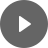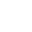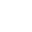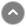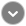Home » Learning Multiplication is More than Memorization!

# Learning Multiplication is More than Memorization!

I have a vivid memory of learning multiplication in third grade.  My teacher, Mrs. Bowman, drew three circles on the chalkboard.  Then she put five milk bottles in each one.  She said this is 3 x 5 which is 15 milk bottles.

That’s really all I remember about learning multiplication, but for some reason, it stuck!  I also remember using my Pee-Chee folder to look up the multiplication tables for dividing in fourth grade.  I think that is how I memorized them.  But times have changed.

With today's Common Core State Standards, multiplication is more than just memorizing the tables.Click To Tweet

UPDATE: November 2020

STOP! With the switch to distance learning for many teachers and students, I wrote another blog post with ideas for teaching multiplication virtually. You can see it HERE!

## How is Learning Multiplication Today Different?

Today, students have to understand the relationship between addition and multiplication as well as multiplication and division.  They have to understand multiplication as equal groups that can be modeled with objects or arrays.  Students need to understand how to use the Properties of Multiplication.  And yes, students need to fluently multiply within 100. So that means that learning multiplication is more than memorization.

In this blog post, I'll show you how I take my students from learning multiplication to attaining multiplication fluency by the end of the year.Click To Tweet

Warning! This is a long post.  Why? Because it explains all the parts that go together over a year-long approach to teaching multiplication.

## Why is Skip Counting Important for Learning Multiplication

I start with skip counting.  Most students by third grade can skip count by 2s, 3s, 5s, and 10s. There are many ways to practice this.

• skip counting songs
• videos
• counting bundles of objects
• choral chants
• poems

Why is it important?  Part of having multiplication fluency is automaticity.  Being able to quickly recall.  Skip counting can build part of that automaticity when learning multiplication.  Skip counting doesn’t have to be done during a math block.  When you have a few minutes between subjects or need to fill in some time, try some skip counting.  Or make it part of your math routine as a warm-up for the first 3-5 minutes.

## Learning the Squares

What? What squares, you ask?  A square is a factor that is multiplied by itself:  3 x 3, or 4 x 4.  Why learn these?  If you exclude 0 and 1, then there are only 11 squares to memorize (2 x 2 up to 12 x 12).

Have your students memorize the multiplication squares because later on, it will help them with a fluency strategy.Click To Tweet

## Why are Equal Groups Important for Learning Multiplication?

First up, is to teach the concept of multiplication as equal groups.  Why?  Equal groups are related to repeated addition and arrays.  I started the lesson with a Multiplication PowerPoint  that I created specifically for my third graders.  It is actually 3 lessons (Lesson 1 is Equal Groups, Lesson 2 is Arrays, and Lesson 3 is number lines).

I created this PowerPoint because I needed a way to explicitly demonstrate these concepts in an engaging and fun way.  This PowerPoint is animated, has sound, questions to generate mathematical thinking and partner sharing.  But most importantly, it integrates a hands-on learning approach with manipulatives.

Once I introduce the concept of equal groups it’s time to practice with some hands-on math.  I’m a big believer that children need to work on a concrete level of mathematics before even attempting to understand the symbols and numbers that are attached to abstract reasoning.  In the PowerPoint, there are specific slides that engage the students with hands-on math.

## Hands-on Time for Learning Multiplication

I instructed the students to take out 12 tiles.  I demonstrated how to put them into equal groups of 6. Then I had them practice putting the same 12 tiles into groups of 4, then 3, and 2.   Each student had a dry erase marker to draw the circles on their desk around the groups so they could visually see the groups.

From there we practiced using the vocabulary:  3 groups of 4, 6 groups of 2, 4 groups of 3, etc.  When I could determine that the majority of the class understood equal groups, we practiced with a printable I created to go along with the PowerPoint.

Students drew a model for a particular multiplication sentence using equal groups.  Then, they answered questions about their model. Click To Tweet

I collected the printable so I could quickly assess student understanding of equal groups.  The students also worked on similar problems in their consumable math book.  The homework also followed up on this concept of equal groups.

The next day I reviewed equal groups again.  Then it was time to introduce the concept of repeated addition.  By the way, there is controversy about whether we should even teach multiplication as repeated addition!  But don’t panic, I would say yes, go ahead and teach it as repeated addition.  You can read the linked article to see why there is a controversy about this!

UPDATE 2020!

So I posed a problem for them:  Looking at the equal groups on your desk, how could you quickly find the total without skip counting?  Eventually, we talked about how we could just add each group together (3 + 3 + 3 + 3 = 12).   What is the purpose of this?  It’s to get kids to see multiplication from many perspectives:  what it is and what it isn’t.

For example, if you had 3 groups of 2 and 1 group of 3, would that be multiplication?  Some would say yes and some would say no.  No, because the groups aren’t equal.  Yes, because you can multiply the equal groups and then add the unequal group.  The point is, addition is related to multiplication and the students need to know that!

## How to Use Arrays to Teach Multiplication

On a different day, I present a problem on the whiteboard such as the one below.  With that, I instruct the students to use the foam tiles to solve the problem in any way they could.  As this was happening, I would search for students who used equal groups and send them to the board to copy their solution below the problem.

I looked for other students who also used equal groups but put the groups in a different way to go up to the board to copy their solution.  Finally, if any of the students lined up their tiles in an array, I would have that student go up and copy it, too!

Once I had several solutions, we came back as a class and I had each of those students explain how they arrived at their solution.

The other students had to listen because they knew I would use the Math Talk prompts

• who can repeat that?
• anyone else can add to that?
• who can explain it a different way?
For the student who made an array, I had also taken a video with my phone of him making the array.  I would share with the class.Click To Tweet

In this video, you’ll see the student constructing an array for a different problem.

## Student Explains How He Used an Array

But first, I had him come up and explained what he did.   Would you believe me if I told you that he actually used the term rows!  Yes, he did!  I said I had to record his thinking on the board so I wrote:  4 tiles in 3 rows.  I explained to my class that this student just discovered another way to multiply:  arrays!

At this point, I explained that arrays are groups but they are formed with equal rows and columns and have a rectangular or square shape.

I continued with lesson 2 of the Multiplication PowerPoint  I created:  using arrays.  We continued to practice forming arrays to match a multiplication sentence.  Once I knew the students had gotten it, I had the work with a partner on a more complicated multiplication fact such as 8 x 6 which required making a larger array.

Working as partners, they could quickly assemble the array and produce a product.  Finally, I assigned them independent work from the consumable which you see below.

## Using a Number line for Learning Multiplication

You would think that such a great visual that has been used since first grade and maybe even kinder, would work wonderfully for learning multiplication.  Nope.  Using a number line is tricky and confusing for kids.

What difficulties would they encounter?

• not starting at zero
• not skip counting correctly
• counting the starting number as part of the skip counting
• confusing “jumps” with “how many to jump”

In my instruction, I gave each student these wonderful and colorful number lines which I laminated. I like the fact they only go up to 30, which is a good number when dealing with multiplication.  But before that, I started with Lesson 3 of the Multiplication PowerPoint I created.  Through animation, it shows how to jump on a number line to multiply

I taught them these steps when we practiced with whiteboards.

Example:  for 3 groups of 4 or 3 x 4

1. Circle the first number in the multiplication sentence.  This is the number of jumps.
2. Underline the second number in the multiplication sentence.  This is how much to jump.
3. Put a dot on the zero.
4. Jump to the number 4 (as in this example of 3 x 4) and put a dot.
5. Then draw an arched line back to the zero.  Label it with 1.
6. Then count ahead another 4 spaces or lines and put a dot.
7. Draw an arched line back to the previous dot, label it with a 2
8. Continue the process until you have 3 jumps (as in this example of 3 x 4).
9. What is the final number?
By following these steps, a lot of the confusion is cleared up and students used the number line properly for learning multiplication. Click To Tweet

I prefer to sometimes use the consumable Go Math book to save on copying paper.  I hand pick which problems the students would work on.

## Properties of Multiplication for Learning Multiplication

So what is left to teach for learning multiplication?  The Properties of Multiplication!  These properties are very important for learning multiplication.  Why?  Because they make learning the multiplication facts easier!

Because of the Zero and Identity Properties of Multiplication, we know that any factor multiplied by zero is zero, while any factor multiplied by 1 is that factor.  But the most important property is the Commutative Property.  Knowing that 7 x 4 produces the same product as  4 x 7 reduces the number of facts to memorize when you take into account the entire multiplication chart.

Watch this YouTube video that explains how the entire multiplication chart can be brought down to 6 facts to memorize.

## What Resources Do I Use to Teach the Properties of Multiplication?

I also have developed a PowerPoint for the Properties of Multiplication (Zero, Identity, Commutative and Associative Properties).

I also have some follow-up activities to reinforce those properties:  Properties of Multiplication Practice.

These reinforcement activities include:

• Bookmark to use as a study guide
• Flap book with the definitions and examples of the properties
• Mini-book of the properties
• Venn Diagrams to compare the properties
• Commutative Property Match Up Center
• and Multiplication Hero!

The last one has been a favorite of my students.  It uses this page-sized X for multiplication.  On it, the student writes the definition of each of the properties and an example.  Then the X is cut out and taped to the student’s chest (one piece of masking tape folded over is enough).

To play Multiplication Hero, two students face each other.  One student reads a definition or gives an example of one of the properties by reading his partner’s X that is taped to his/her chest.  The other student has to name the property.  If the answer is correct, they cross their arms across their chest and say multiplication hero!  They continue to switch partners.  This is a fun game to play for a review.

## Are We Done Yet? No!

I’ve taught my students to skip count, the multiplication squares, models for multiplication (equal groups, arrays and number lines),  and the Properties of Multiplication.  So what is left to do?  Eventually, all students do have to memorize the multiplication facts.

I use various approaches for the students to master the facts.  One is to hold them accountable for studying.  Learning to memorize is an important life skill. Click To Tweet

The act of focusing on something for a length of time until it becomes committed to long-term memory requires discipline and good habits.

At Back to School Night, I give each parent a folder with my Multiplication Homework Activity Chart.  I once again remind the parents during parent conferences that this homework will now begin.  I explain how the chart works.  Essentially, the student must complete 3 activities (or more) per week to make a tic-tac-toe.

The activities are varied with different approaches to learning the facts.  I provide copy masters to make flash cards, paper dice, pre-made recording sheets and links to many multiplication websites.  The students do this as weekly homework for 6 months.

Learning the facts is self-paced and the chart provides many different ways to memorize them.  This resource comes with everything you need to get your students studying the multiplication facts.  There is also a bilingual English-Spanish version as well.

## Tips and Tricks for Learning the Multiplication Tables

But that isn’t usually enough.  I also teach my students strategies or tips for each multiplication table.  For example, doubling the 2s will give you the four times table.  Example:  4 x 6 is like 2 x 6 doubled.  2 x 6 = 12, then double it and you get 24!  The same trick works for the 3s and 6s.

I have created a Multiplication Tips and Strategies Chart of these tips and strategies to give to each student which they put in their math folder.  An additional copy also goes home.

I turned the chart into Multiplication Tips and Strategies Posters that also hang on my math board for reference.

You can try out the Multiplication Tips and Strategies Posters SAMPLER here.

## I Provide Practice Time in Class

Usually, around the beginning of December when I start teaching division, I notice that some students are stuck on a particular multiplication table. That is when I bring out the Multiplication Practice Cards for select students.

I already have these stored in baggies with a dry erase marker and felt eraser.  I give the student the set for a particular multiplication table and they take it home to study.  When I started this process last year, it helped jump-start those kids that were stuck.

## My Ah-Hah Moment!

Usually, around late January or early February, I started noticing that students who previously learned a complete multiplication table now couldn’t remember it.  Not with all the tables, but mostly with the 3s, 4s, 6s, 7s, 8s.  Obviously, memorization is NOT enough.

Fluency does not mean automatic recall. Fluency is many faceted. If you can't remember, what strategy can you use to quickly figure it out?  So fluency is a mix of automaticity, recall and use of strategies.Click To Tweet

That is when I discovered the multiplication fluency strategies.  These are the missing link to multiplication fluency.  Now, don’t panic.  If you’ve taught skip counting, the squares and the Properties of Multiplication, then you’ve taught about half of the strategies already!

## Don’t Forget to Teach the OTHER Half of the Strategies!

The other half of the strategies involve teaching

• halving and doubling
• subtracting a group
• using a square
• using the distributive property

These are the strategies when combined with the previous teaching will have your students multiplying fluently by the end of the year.

You can read more about these strategies on this blog post:  Frustrated That Students Don’t Know the Multiplication Facts?

Here’s a video of halving and doubling strategy being used:

At first, students practice these strategies with paper and pencil (or laminated templates and dry erase markers). Once they get some practice, we try to do some example as mental math.  Using these strategies as mental math strategies is the ultimate goal.

I’d like to offer you this FREE Guide to Achieving Multiplication Fluency that explains all the strategies while giving you suggestions for teaching these strategies to your students.  Just by signing up below for my Newsletter, I’ll send you a link to the Guide to Achieving Multiplication Fluency.

You will see that these strategies will provide the missing link to attaining multiplication fluency.  These strategies can be introduced in the second half of the year as a boost to get your students to multiplication fluency.

Here’s another resource I created to make teaching these strategies easier for you.  This resource was made to save you time and effort in creating all the materials needed to support these strategies.

The resource comes with teaching posters, teaching suggestions, games for practice, practice templates, practice pages and more.  If you sign up for my Newsletter to get the FREE Guide to Achieving Multiplication Fluency I’ll also send you some information on how to get the Multiplication Fluency Resources package at a discount!

# Don’t Go Yet!

Are you new to the 1:1 classroom setting? Then you’ll want to read my Valuable Tips for the 1:1 Classroom.

Check out how I use Google Classroom to present at Back to School Night for Parents.###### Follow:

Hi, I’m Claudio, the dad from Two Boys and a Dad. Whether you’re just starting out teaching or a veteran teacher, let me offer you some ideas, tips, suggestions, resources and a sounding board for your daily classroom struggles (and successes!). This is your place to find information and ask questions about teaching in the elementary grades.

Share: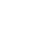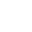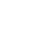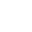### Interactive Digital Notebooks for Google Slides®